# 神经网络提取PDF表格工具来了，支持图片，还能白嫖谷歌GPU资源

“表哥表姐”们还在为大量PDF文件中的表格发愁吗？

“表哥表姐”们还在为大量PDF文件中的表格发愁吗？

# 测试实例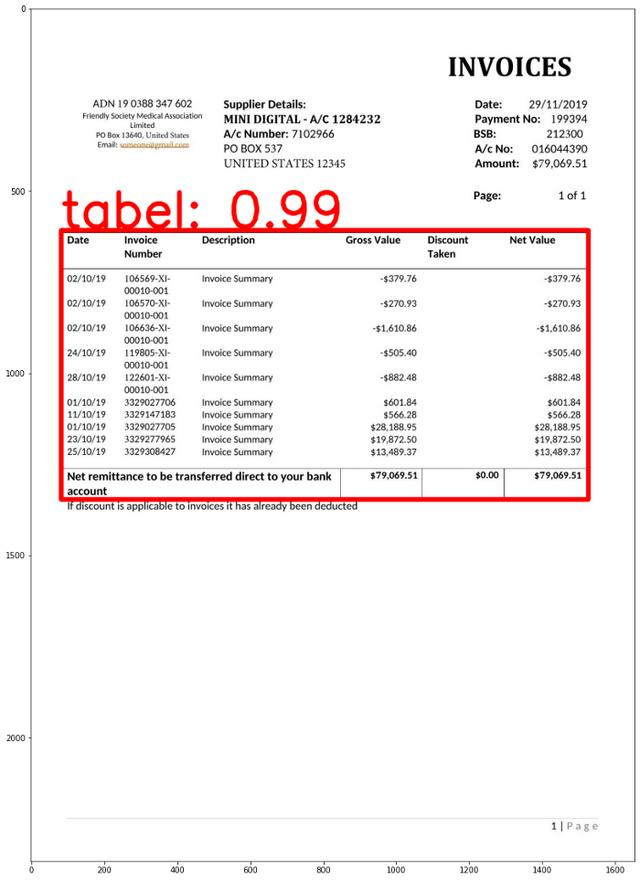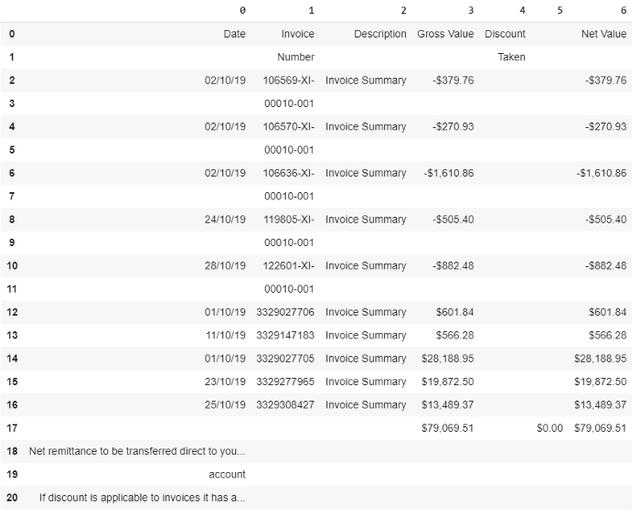# 使用姿势详解

``git clone https://github.com/fizyr/keras-retinanet``

``pip install .python setup.py build_ext — inplace``

# 训练Keras-RetinaNet识别表格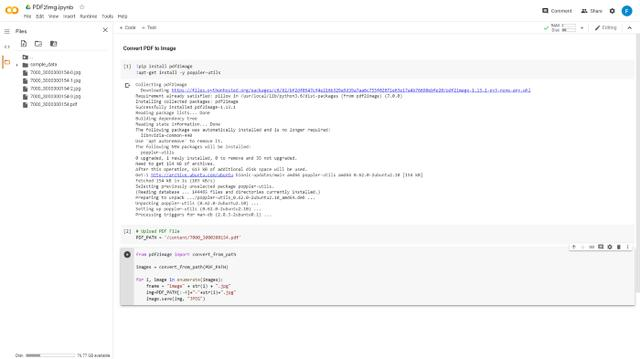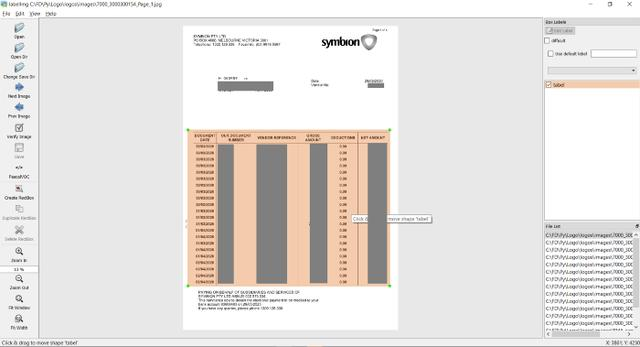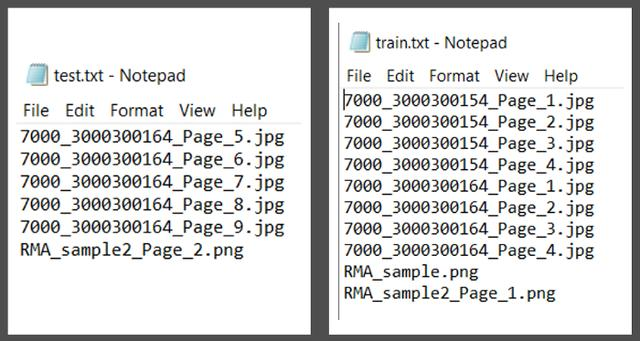``python build_logos.py``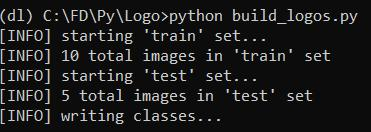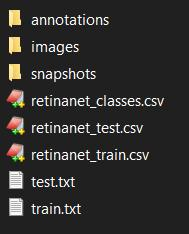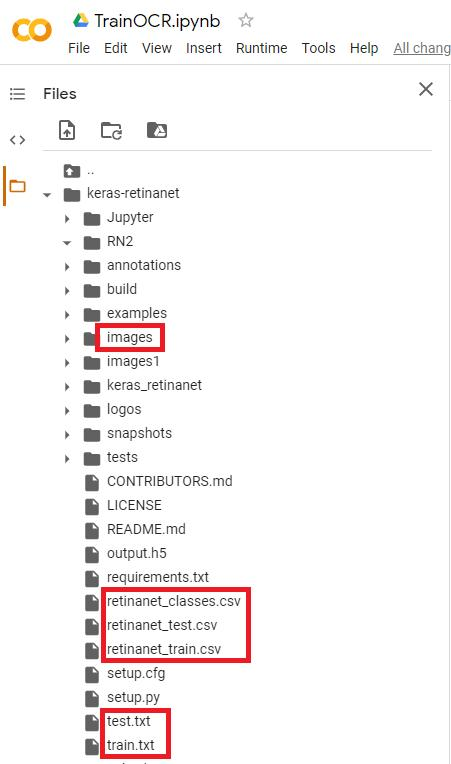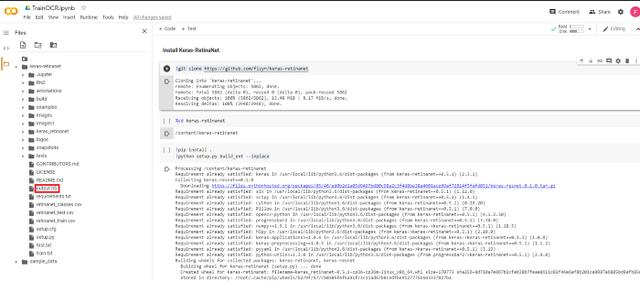# 运行测试

PyPDF2是一个python工具库，能够提取文档信息，裁剪页面等。

``pip install PyPDF2``

Camelot是专门用于解析PDF页面表格的python库。

``pip install camelot-py[cv]``

PDF2IMG是将Python转换为PIL Image对象的python库。

``pip install pdf2imag``

``model_path = ‘output.h5’model = models.load_model(model_path, backbone_name=’resnet50’)labels = ‘retinanet_classes.csv’LABELS = open(labels).read().strip().split(‘\n’)LABELS = {int(L.split(‘,’)): L.split(‘,’) for L in LABELS}print(LABELS){0: ‘tabel’}``

``import numpy as npimport cv2import matplotlib.pyplot as pltimage_path = imgfname#image = cv2.imread(image_path)image = read_image_bgr(image_path)image = cv2.cvtColor(image, cv2.COLOR_BGR2RGB)output = image.copy()output = cv2.cvtColor(output, cv2.COLOR_BGR2RGB)print(output.shape) # row (height) x column (width) x color (3)image = preprocess_image(image)(image, scale) = resize_image(image)image = np.expand_dims(image, axis=0)# detect objects in the input image and correct for the image scale(boxes, scores, labels) = model.predict_on_batch(image)boxes /= scaleconfidence =  0.2from google.colab.patches import cv2_imshowimport matplotlib.pyplot as pltlabel_out = []result = ""# loop over the detectionsfor (box, score, label) in zip(boxes, scores, labels):  # filter out weak detections  if score < confidence:    continue  # convert the bounding box coordinates from floats to integers  box = box.astype("int")  # build the label and draw the label + bounding box on the output  # image  labeli = label  label = "{}: {:.2f}".format(LABELS[label], score)  print(label)  if LABELS[labeli] not in label_out:      label_out.append(LABELS[labeli])      cv2.rectangle(output, (box, box), (box, box), (255, 0, 0), 12)      print(box)      print(box)      print(box)      print(box)      #result            cv2.putText(output, label, (box, box - 10), cv2.FONT_HERSHEY_SIMPLEX, 4.5, (255, 1, 1), 12)plt.figure(figsize=(20, 20))plt.imshow(output)plt.show()````interesting_areas=[]output = [[x1, y1, x2, y2]]for x in output:  [x1, y1, x2, y2] = bboxes_pdf(img, pdf_page, x)  bbox_camelot = [            ",".join([str(x1), str(y1), str(x2), str(y2)])        ]  # x1,y1,x2,y2 where (x1, y1) -> left-top and (x2, y2) -> right-bottom in PDF coordinate space        #print(bbox_camelot)  interesting_areas.append(bbox_camelot)print(interesting_areas)output_camelot = camelot.read_pdf(    filepath=pdf_file, pages=str(pg), flavor="stream", table_areas=interesting_areas)output_camelot.df``# 图片怎么办

Ocrmypdf是一个python软件包，可将基于图像的pdf转换为基于文本的PDF。

``brew install ocrmypdf``

``ocrmypdf input_file.pdf output_file.pdf``

# 传送门

https://github.com/ferrygun/PDFTableExtract

https://www.makesense.ai/